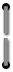# Applications

## Investments

The following applications use the financial keys pressed in left to right order to obtain solutions. Some of the applications use interactive and basic keys also. As in ordinary tables, the number of years and interest rate must be converted to reflect the number of compounding periods if different from those specified. When converting years to periods, multiply the years by the number of compounding periods per year, and load the result by pressingn. When converting an interest rate to its periodic equivalent, divide the rate by the number of compounding periods per year and load the result by pressingi. Then proceed with the calculation.

### Compounded Amounts

#### Future Value of Compounded Amount

This calculation finds the future value of an amount compounded at a certain interest rate over a specified number of periods. Information is entered as follows:1Enter number of time periods, pressn.2Enter rate per period, pressi.3Enter principal (present value), pressPV.4PressFVto obtain the future value.
Sample Case 1: What is the future value of \$500 invested at 6% for 7 years if interest is compounded annually?
Solution
Enter:  See Displayed:

 7n6i500PVFV\$751.82
value in 7 years compounded annually
Sample Case 2: What is the future value of the same \$500 invested at the same rate (6%) for the same number of years (7) if compounded quarterly? The necessary intermediate calculations can be done in your head or as follows on the HP-80:25